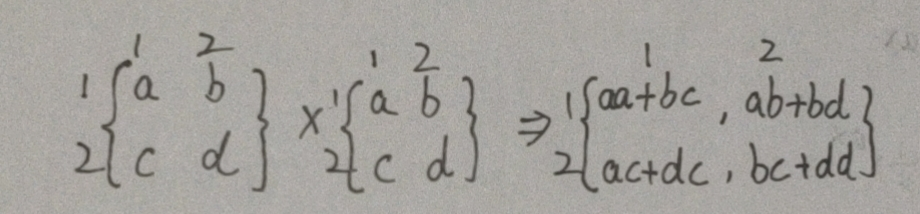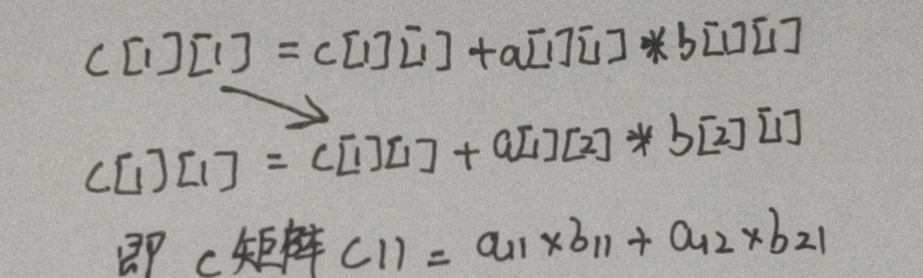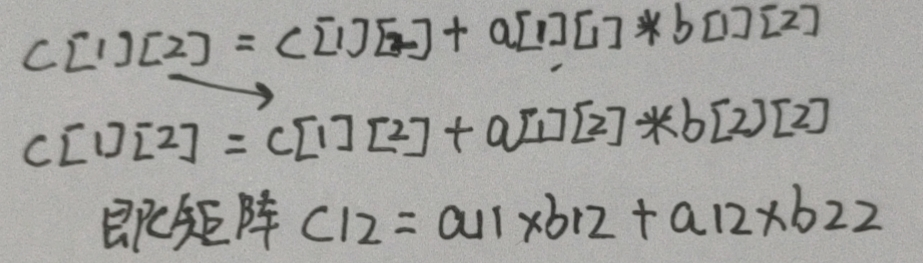# 从频度引发的c语言多重for循环乃至编写算法思路的思考

2023-09-24 9 0

• 频度
• 矩阵相乘过程
• 思考算法的设计原理
• 进行算法设计

## 频度

``for (i=1;i<=n;i++)    //频度为n+1for(j=1;j<n;j++)   //频度为n*(n+1){c[i][j]=0;        //频度为n^2for(k=1;k<=n;k++) //频度为n^2*(n+1)c[i][j]=c[i][j]+a[i][k]*b[k][j]; //频度为n^3}``

`for (i=1;i<=n;i++) //频度为n+1`

`for(j=1;j<n;j++) //频度为n*(n+1)`

1.因为第一个for循环实际执行了n次，所以传递给第二个for循环n次。
2.类比第一个for循环，第二个for循环实际执行次数是n次，但最后一次碰壁了，所以总共执行了n+1次
3.总共就是n*(n+1)次

…别问我为什么不是n+(n+1)次，如果有这样疑问的同学需要重新学习一下c语言

`c[i][j]=0; //频度为n^2`

`for(k=1;k<=n;k++) //频度为(n+1)n^2`

1.从前两个for循环上得到了n*n次的循环，所以是n^2
2.类似第一个循环，总共执行了（n+1）次

`c[i][j]=c[i][j]+a[i][k]*b[k][j]; //频度为n^3`

## 矩阵相乘过程

``````c[i][j]=c[i][j]+a[i][k]*b[k][j];
``````①第一个for循环传递i=1，i<=2，为什么小于2？后面有解释
②第二个for循环传递j=1，j<=2
`c=0`
④第三个for循环传递k=1，K<=2
`c=c+a*b`
⑥第三个for循环传递k=2
`c=c+a*b //这里的右值c是第5个步骤里的结果`①第一个for循环还是1
②第二个for循环传递j=2
`c=0`
④第三个for循环传递k=1
`c=c+a*b //注意，b的第二个元素从1变成了2`
⑥第三个for循环传递k=2
`c=c+a*b //这里的右值c是第5个步骤里的结果`c[i][j]最大就是c

## 思考算法的设计原理

``````c[i][j]=0;
``````

``````c[i][j]=c[i][j]+a[i][k]*b[k][j];
``````

A的第二个元素=B的第一个元素=K

## 进行算法设计

``````c[i][j]=a[i][k]+b[k][j]
``````

``````for(K=1;K<=2;K++)
``````

C矩阵一开始是0，所以设计

``````c[i][j]=0
``````

C的四个元素是C11,C12,C21,C22

``````for(i=1;i<=2;i++)for(j=1;j<=2;j++)
``````

2021年6月28日 00:06:21